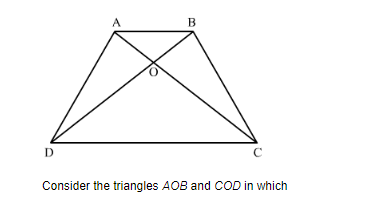# ABCD is a trapezium having AB || DC. Prove that O,

Question:

$A B C D$ is a trapezium having $A B \| D C$. Prove that $O$, the point of intersection of diagonals, divides the two diagonals in the same ratio. Also prove that $\frac{\operatorname{ar}(\Delta O C D)}{\operatorname{ar}(\Delta O A B)}=\frac{1}{9}$, if $A B=3 C D$.

Solution:

We are given ABCD is a trapezium with AB||DC$\angle A O B=\angle C O D$

$\angle A B O=\angle O D C$(alternative angle)

$\angle B A O=\angle D C A$(alternative angle)

Therefore, $\triangle O D C \sim \triangle O B A$

$\Rightarrow \frac{A O}{O C}=\frac{B O}{D O}=\frac{A B}{C D}$

$\Rightarrow \frac{A O}{O C}=\frac{B O}{D O}$

Hence we have proved that O, the point of intersection of diagonals, divides the two diagonals in the same ratio.

We are given $A B=3 C D$ and we have to prove that $\frac{\operatorname{ar}(\Delta O C D)}{a r(\Delta O A B)}=\frac{1}{9}$

We already have proved that AOB and COD are similar triangles

So

$\frac{a r(\Delta O C D)}{a r(\Delta O A B)}=\frac{C D^{2}}{A B^{2}}$

$\frac{a r(\Delta O C D)}{a r(\Delta O A B)}=\frac{C D^{2}}{(3 C D)^{2}}$

$\frac{\operatorname{ar}(\Delta O C D)}{\operatorname{ar}(\Delta O A B)}=\frac{1}{9}$

Hence, Prove that $\frac{\operatorname{ar}(\Delta O C D)}{\operatorname{ar}(\Delta O A B)}=\frac{1}{9}$